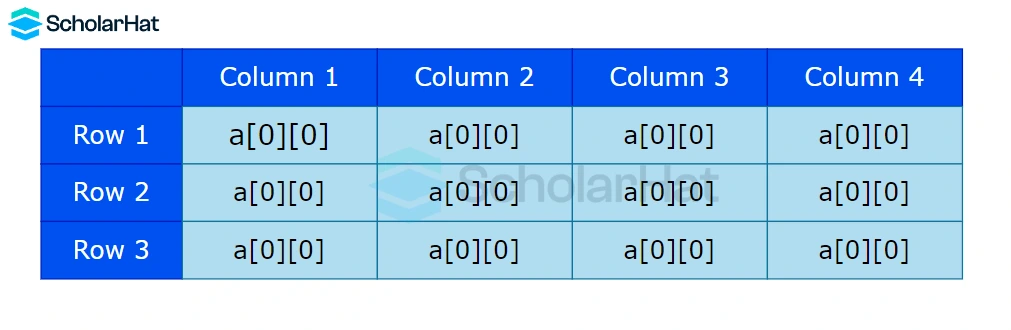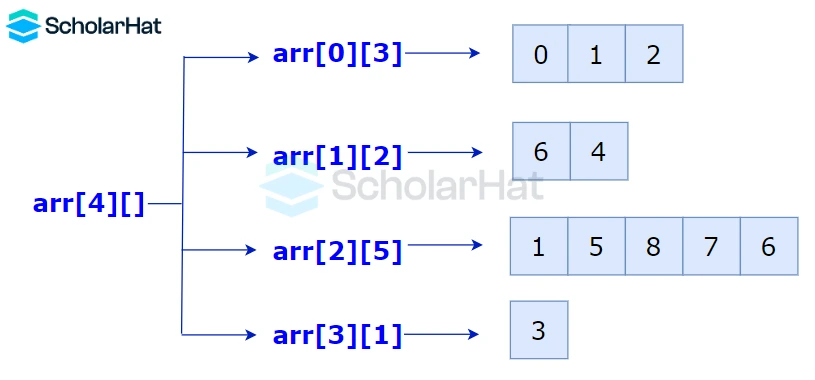# Types of Arrays in C#: Single-dimensional, Multi-dimensional and Jagged Array

16 Nov 2023
Intermediate
221 ViewsLearn via Video Course & by Doing Hands-on Labs

## Types of Array in C#: An Overview

Welcome to our comprehensive guide on the various types of arrays in C#. Arrays are fundamental data structures used to store elements of the same data type sequentially. In this article, we'll explore the different types of arrays in C#, their features, and how to effectively utilize them in your programming endeavors

## Types of Arrays in C#

There are three types of arrays in C#, which are

1. Single-dimensional Array in C#
2. Multi-dimensional Array in C#
3. Jagged Array in C#

1. Single-Dimensional Arrays

In C#, a single-dimensional array is a data structure that stores elements of the same data type in a linear sequence. Each element in the array is accessed by its index, starting from zero. Single-dimensional arrays are versatile and commonly used for various programming tasks, providing efficient data storage and retrieval.Declaring Single dimensional array

``````int[] arr = new int;
double numbers = new double;
string[] names = new string;``````

#### Initializing Single dimensional array

``````int[] numbers = new int[] { 1, 2, 3, 4, 5, 6,7,8,9,10 };
string[] names = new string[] { "Roy", "Shiv", "Tara", "Urmi", "Luna", "Samay" };``````

Let’s see the practical implementation of Single-Dimensional Arrays in the following example:

#### Example

``` ```
using System;
class Program
{
static void Main()
{
// Declare and initialize an array
int[] numbers = { 10, 20, 30, 40, 50 };

// Output array elements
Console.WriteLine("Array elements:");
for (int i = 0; i < numbers.Length; i++)
{
Console.WriteLine(numbers[i]);
}
}
}``````

#### Explanation

This C# code initializes an integer array "numbers" with values {10, 20, 30, 40, 50} and then iterates through the array using a for loop to print each element to the console. It demonstrates basic array declaration, initialization, and traversal in a console application.

#### Output

``````Array elements:
10
20
30
40
50``````

### 2. Multi-Dimensional Arrays

Multi-dimensional arrays in C# are arrays that hold data in more than one dimension, allowing for efficient storage and manipulation of complex data structures. Unlike one-dimensional arrays, these arrays can be thought of as matrices or tables, providing rows and columns to organize and access elements based on multiple indices.#### Declaring Multi-dimensional Array

``````string[,] names = new string[5, 5];
int[,] numbers = new int[7, 8];``````

### Multi-dimensional Array Initialization

``````int[,] numbers = new int[4, 3] {{2,3}, {4,5}, {6,7}};
string[,] names = new string[2, 2] {{"Urmi", "Rumi"}, {"Ram", "Bheem"}};``````

Let’s see the practical implementation of Multi-Dimensional Arrays in the following example:

#### Example

``` ```
using System;

class Program
{
static void Main()
{
// Define a 2D array
int[,] myArray = new int[3, 4] {
{1, 2, 3, 4},
{5, 6, 7, 8},
{9, 10, 11, 12}
};

// Get the dimensions of the array
int rows = myArray.GetLength(0);
int columns = myArray.GetLength(1);

// Display the array elements
Console.WriteLine("Array elements:");
for (int i = 0; i < rows; i++)
{
for (int j = 0; j < columns; j++)
{
Console.Write(myArray[i, j] + " ");
}
Console.WriteLine();
}
}
}``````

#### Explanation

This code defines a 2D array called myArray with 3 rows and 4 columns. It initializes the array with specific values. Then, it retrieves the dimensions of the array using the GetLength method. The code then uses nested loops to iterate over the array and prints each element on the console. The output displays the array elements in a tabular format, with each row on a separate line.

#### Output

``````Array elements:
1 2 3 4
5 6 7 8
9 10 11 12``````

### 3. Jagged Array in C#

In C#, a jagged array is an array of arrays where each element can hold arrays of different sizes. Unlike regular multi-dimensional arrays, jagged arrays allow for flexible and uneven lengths, making them ideal for storing complex data structures like tables or matrices with varying row lengths.#### Declaring and initializing Jagged array.

``````int[][] jaggedArray = new int[];
jaggedArray = new int;
jaggedArray = new int;
jaggedArray = new int[] { 4, 6, 8, };
jaggedArray = new int[] { 1, 0, 2, 4, 6 };
``````

You can also initialize the array upon declaration like this:

``````int[][] jaggedArray = new int[][]
{
new int[] { 4, 6, 8, },
new int[] { 1, 0, 2, 4, 6 }
};``````

You can use the following shorthand form:

``````int[][] jaggedArray =
{
new int[] { 4, 6, 8, },
new int[] { 1, 0, 2, 4, 6 }
};``````

Let’s see the practical implementation of Jagged Arrays in the following example:

#### Example

``` ```

using System;class Program
{
static void Main()
{
// Create a multi-dimensional jagged array
int[][] jaggedArray = new int[]
{
new int[] { 1, 2, 3 },
new int[] { 4, 5 },
new int[] { 6, 7, 8, 9 }
};

// Display the elements of the jagged array
for (int i = 0; i < jaggedArray.Length; i++)
{
Console.Write("Row {0}: ", i);
for (int j = 0; j < jaggedArray[i].Length; j++)
{
Console.Write(jaggedArray[i][j] + " ");
}
Console.WriteLine();
}
}
}``````

#### Explanation

This code defines a 2D array called myArray with 3 rows and 4 columns. It initializes the array with specific values. Then, it retrieves the dimensions of the array using the GetLength method. The code then uses nested loops to iterate over the array and prints each element on the console. The output displays the array elements in a tabular format, with each row on a separate line.

#### Output

``````Row0:123
Row1:45
Row2:6789``````
##### Conclusion

In conclusion, understanding the various types of arrays in C# is essential for efficient programming. Whether it's a single-dimensional array, multi-dimensional array, or jagged array, each type has its unique applications. Mastering these concepts empowers developers to optimize their code and solve complex problems effectively.

## Take our free csharp skill challenge to evaluate your skill

In less than 5 minutes, with our skill challenge, you can identify your knowledge gaps and strengths in a given skill.

Share Article
Similar Articles